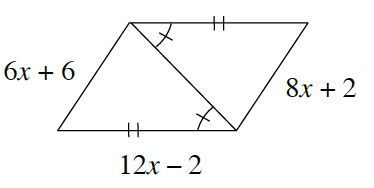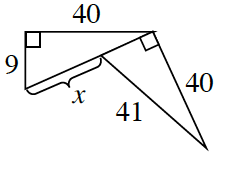Home > CCG > Chapter Ch7 > Lesson 7.2.4 > Problem7-86

7-86.

For each figure below, determine if the two smaller triangles in each figure are congruent. If so, create a flowchart to explain why. Then, solve for $x$. If the triangles are not congruent, explain why not. Redraw and label the diagrams as needed.

Each set of triangles is congruent. Now show why or how.

1.Congruent because of $\text{SAS}≅$, $x=2$.

1.Congruent because of $\text{HL}≅$, $x=32$.